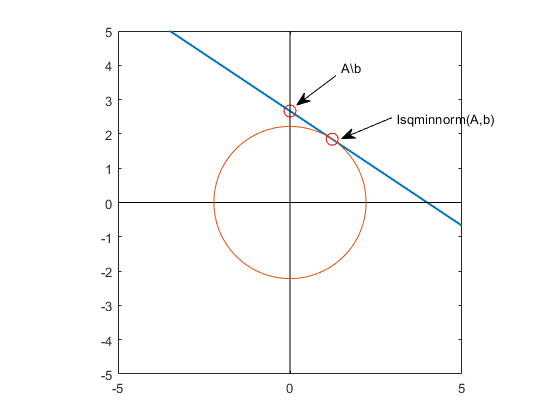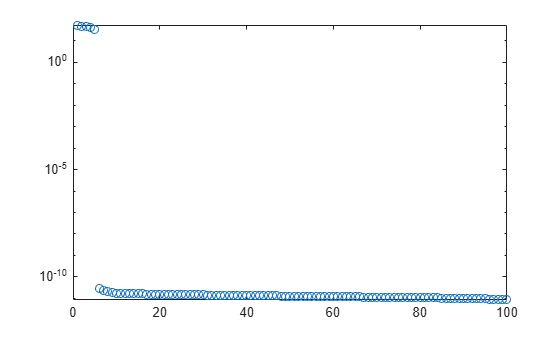Documentation

# lsqminnorm

Minimum norm least-squares solution to linear equation

## Syntax

``X = lsqminnorm(A,B)``
``X = lsqminnorm(A,B,tol)``
``X = lsqminnorm(___,rankWarn)``

## Description

example

````X = lsqminnorm(A,B)` returns an array `X` that solves the linear equation AX = B and minimizes the value of `norm(A*X-B)`. If several solutions exist to this problem, then `lsqminnorm` returns the solution that minimizes `norm(X)`.```

example

````X = lsqminnorm(A,B,tol)` additionally specifies the tolerance that `lsqminnorm` uses to determine the rank of `A`.```

example

````X = lsqminnorm(___,rankWarn)` specifies an optional flag to display a warning if `A` has low rank. You can use any of the input argument combinations in previous syntaxes. `rankWarn` can be `'nowarn'` (default) or `'warn'`.```

## Examples

collapse all

Solve a linear system that has infinitely many solutions with backslash (`\`) and `lsqminnorm`. Compare the results using the 2-norms of the solutions.

When infinite solutions exist to $\mathrm{Ax}=\mathit{b}$, each of them minimizes $‖\mathrm{Ax}-\mathit{b}‖$. The backslash command (`\`) computes one such solution, but this solution typically does not minimize $‖\mathit{x}‖$. The solution computed by `lsqminnorm` minimizes not only `norm(A*x-b)`, but also `norm(x)`.

Consider a simple linear system with one equation and two unknowns, $2{\mathit{x}}_{1}+3{\mathit{x}}_{2}=8$. This system is underdetermined since there are fewer equations than unknowns. Solve the equation using both backslash and `lsqminnorm`.

```A = [2 3]; b = 8; x_a = A\b```
```x_a = 2×1 0 2.6667 ```
`x_b = lsqminnorm(A,b)`
```x_b = 2×1 1.2308 1.8462 ```

The two methods obtain different solutions because backslash only aims to minimize `norm(A*x-b)`, whereas `lsqminnorm` also aims to minimize `norm(x)`. Calculate these norms and put the results in a table for easy comparison.

```s1 = {'Backslash'; 'lsqminnorm'}; s2 = {'norm_Ax_minus_b','norm_x'}; T = table([norm(A*x_a-b); norm(A*x_b-b)],[norm(x_a); norm(x_b)],'RowNames',s1,'VariableNames',s2)```
```T=2×2 table norm_Ax_minus_b norm_x _______________ ______ Backslash 0 2.6667 lsqminnorm 1.7764e-15 2.2188 ```

This figure illustrates the situation and shows which solutions each of the methods return. The blue line represents the infinite number of solutions to the equation ${\mathit{x}}_{2}=-\frac{2}{3}{\mathit{x}}_{1}+\frac{8}{3}$. The orange circle represents the minimum distance from the origin to the line of solutions, and the solution returned by `lsqminnorm` lies exactly at the tangent point between the line and circle, indicating it is the solution that is closest to the origin.Show how specifying a tolerance for the rank computation in `lsqminnorm` can help define the scale of the problem so that random noise does not corrupt the solution.

Create a low-rank matrix of rank 5 and a right-hand side vector `b`.

```rng default % for reproducibility U = randn(200,5); V = randn(100,5); A = U*V'; b = U*randn(5,1) + 1e-4*randn(200,1);```

Solve the linear system $\mathrm{Ax}=\mathit{b}$ using `lsqminnorm`. Compute the norms of `A*x-b` and `x` to check the quality of the solution.

```x = lsqminnorm(A,b); norm(A*x-b)```
```ans = 0.0014 ```
`norm(x)`
```ans = 0.1741 ```

Now add a small amount of noise to the matrix `A` and solve the linear system again. The noise affects the solution vector `x` of the linear system disproportionately.

```Anoise = A + 1e-12*randn(200,100); xnoise = lsqminnorm(Anoise,b); norm(Anoise*xnoise - b)```
```ans = 0.0010 ```
`norm(xnoise)`
```ans = 1.1215e+08 ```

The reason for the big difference in the solutions is that the noise affects the low-rank approximation of `A`. In other words, `lsqminnorm` is treating small values on the diagonal of the `R` matrix in the QR decomposition of `A` as being more important than they are. Ideally, these small values on the diagonal of `R` should be treated as zeros.

Plot the diagonal elements of the `R` matrix in the QR decomposition of `Anoise`. A large number of the diagonal elements are on the order of 1e-10.

```[Q,R,p] = qr(Anoise,0); semilogy(abs(diag(R)),'o')```The solution to this issue is to increase the tolerance used by `lsqminnorm` so that a low-rank approximation of `Anoise` with error less than 1e-8 is used in the calculation. This makes the result much less susceptible to the noise. The solution using a tolerance is very close to the original solution `x`.

```xnoise = lsqminnorm(Anoise, b, 1e-8); norm(Anoise*xnoise - b)```
```ans = 0.0014 ```
`norm(xnoise)`
```ans = 0.1741 ```
`norm(x - xnoise)`
```ans = 1.0811e-14 ```

Solve a linear system involving a low-rank coefficient matrix with warnings turned on.

Create a 3-by-3 matrix that is of rank 2. In this matrix, you can obtain the third column by adding together the first two columns.

`A = [1 2 3; 4 5 9; 6 7 13]`
```A = 3×3 1 2 3 4 5 9 6 7 13 ```

Find the minimum norm least-squares solution to the problem $\mathrm{Ax}=\mathit{b}$, where $\mathit{b}$ is equal to the second column in $\mathit{A}$. Specify the `'warn'` flag for `lsqminnorm` to display a warning if it detects that `A` is of low rank.

```b = A(:,2); x = lsqminnorm(A,b,'warn')```
```Warning: Rank deficient, rank = 2, tol = 1.072041e-14. ```
```x = 3×1 -0.3333 0.6667 0.3333 ```

## Input Arguments

collapse all

Coefficient matrix. The coefficient matrix appears in the system of linear equations on the left as Ax = B. The coefficient matrix can be full or sparse.

Data Types: `single` | `double`
Complex Number Support: Yes

Input array, specified as a vector or matrix. `B` appears in the system of linear equations on the right as Ax = B. If `B` is a matrix, then each column in the matrix represents a different vector for the right-hand side.

Data Types: `single` | `double`
Complex Number Support: Yes

Rank tolerance, specified as a nonnegative scalar. Specifying the tolerance can help prevent the solution from being susceptible to random noise in the coefficient matrix. By default, `lsqminnorm` computes `tol` based on the QR decomposition of `A`.

`lsqminnorm` computes the rank of `A` as the number of diagonal elements in the `R` matrix of the QR decomposition `[Q,R,p] = qr(A,0)` with absolute value larger than `tol`. If the rank of `A` is `k`, then the function forms a low-rank approximation of `A` by multiplying the first `k` columns of `Q` by the first `k` rows of `R`. Changing the tolerance affects this low-rank approximation of `A`.

Example: `X = lsqminnorm(A,B,1e-2)`

Data Types: `double`

Warning toggle for low-rank matrices, specified as either `'nowarn'` or `'warn'`. Specify `'warn'` to indicate that `lsqminnorm` should produce warnings if the coefficient matrix `A` is rank deficient.

Example: `X = lsqminnorm(A,B,'warn')`

## Tips

• The minimum-norm solution computed by `lsqminnorm` is of particular interest when several solutions exist. The equation Ax = b has many solutions whenever `A` is underdetermined (fewer rows than columns) or of low rank.

• `lsqminnorm(A,B,tol)` is typically more efficient than `pinv(A,tol)*B` for computing minimum norm least-squares solutions to linear systems. `lsqminnorm` uses the complete orthogonal decomposition (COD) to find a low-rank approximation of `A`, while `pinv` uses the singular value decomposition (SVD). Therefore, the results of `pinv` and `lsqminnorm` do not match exactly.

• For sparse matrices, `lsqminnorm` uses a different algorithm than for dense matrices, and therefore can produce different results.